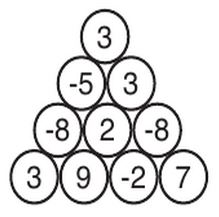Problems

# Ball Stacking

The XYZ TV channel is developing a new game show, where a contestant has to make some choices in order to get a prize. The game consists of a triangular stack of balls, each of them having an integer value, as the following example shows.The contestant must choose which balls he is going to take and his prize is the sum of the values of those balls. However, the contestant can take any given ball only if he also takes the balls directly on top of it. This may require taking additional balls using the same rule. Notice that the contestant may choose not to take any ball, in which case the prize is zero.

The TV show director is concerned about the maximum prize a contestant can make for a given stack. Since he is your boss and he does not know how to answer this question, he assigned this task to you.

Input

Each test case is described using several lines. The first line contains an integer N representing the number of rows of the stack (1 N 1000). The i-th of the next N lines contains i integers Bij (-105Bij105 for 1 j i N); the number Bij is the value of the j-th ball in the i-th row of the stack (the first row is the topmost one, and within each row the first ball if the leftmost one).

The last test case is followed by a line containing one zero.

Output

For each test case output a line with an integer representing the maximum prize a contestant can make from the stack.

Time limit 1 second
Memory limit 64 MiB
Input example #1
4
3
-5 3
-8 2 -8
3 9 -2 7
2
-2
1 -10
3
1
-5 3
6 -4 1
0

Output example #1
7
0
6

Source ACM ICPC Latin America 2011, November 4th-5th, 2011